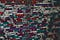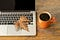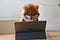# MATLAB 101Photo by Markus Spiske on Unsplash

Let us try to make this a really fast paced, to the point article. Okay? Good. Let’s go!

So, ‘matlab’ is a Hindi language word which translates, in English, to ‘meaning of’. ‘Matlab’ can also be found in the Urdu language and here too it translates to ‘meaning of’.
And this word has got nothing to do with the software MATLAB.
(Haha. Gotcha!)

MATLAB is short for MATrix LABoratory .

It’s case sensitive. The syntax is somewhat like that of C language.

There are four different windows in MATLAB.

1. MATLAB Desktop When you first launch the MATLAB software, what you…

# Mahe’s ‘Operating Systems’ Lessons (MOSLs)Photo by engin akyurt on Unsplash

LESSON TWO

(This is going to be very short.)

An important term in the study of Operating Systems is ‘kernel’.
The kernel is the core of the operating system that has complete control over everything in it. When the computer system is turned on, the kernel of the OS is the first program to load onto the system.
Thus, the kernel facilitates the interactions between hardware and software.

The Dual Mode of Operation of The CPU

It is understood that the CPU operates in two different modes. Namely, the kernel mode and the user mode.

In the kernel mode, the…

# Operating SystemsPhoto by Cookie the Pom on Unsplash

# LESSON ONE

So, you want your computer to do a ‘2+2’ for you (you sucker). You already know that your computer (the hardware, the physical ‘thing’ you can see) is just a machine, made up of metal, plastic, silicon and stuff. You know that your computer (the thing) doesn’t actually understand your language. And you might also know that it has something to do with 1s and 0s. Yes, Binary! That’s its native language. Your computer is from ‘Binaras’.

You know that when your computer does a ‘2+2’, it is actually tweaking those 1s and 0s inside of it and producing the…

# Coin Piles

We are given two piles of coins containing A and B number of coins. In one move we can remove one coin from pile A and two coins from pile B or we can remove two coins from pile A and one from pile B.
(In other words, in one move, coins are removed from both piles and three coins should be removed in total.)
Given the number of coins in each pile, we have to find out whether it is possible to empty both the piles (in any number of moves) or not.

For example: A = 1 coin…

# Creating Strings I

Given a string, the task is to generate all different strings that can be created using it’s characters.

For example, given string = “abc”
All possible distinct strings that can be generated using the three characters in this string are:
1. “abc”
2. “acb”
3. “bac”
4. “bca”
5. “cab”
6. “cba”

Notice that there were three characters in the string, so that possible number of ways in which these three characters could be rearranged is 3! = 6. We fix one character and then insert the remaining two characters to form one combination, and for the next combination we…

# Trailing Zeros

Given an integer ’n’, we are supposed to find out the number of trailing zeros in ‘n!’ .

One obvious way is to calculate n! and count the number of trailing zeros.
But this approach is not efficient as the value of n! can exceed the limit of unsigned long long for 1<n<10⁹ . Hence, we try to device some other method to count the number of trailing zeros in n! without actually having to calculate n!.

Take any number. Let’s say ‘7’.
Let us multiple it by ‘2’.

7*2 = 14

Does multiplying ‘7’ by ‘2’, give us an…

# Palindrome Reorder

Given a string, the task is to rearrange the letters in such a way that the string becomes a palindrome.

Example: AAAABB
After rearranging, the string becomes: AABBAA

Observe that there are four ‘A’s and two ‘B’s in the original string.
The length of the string is six.
Imagine the string being divided into two halves:
AAB + BAA
Clearly the the right half is a inverted version of the left half.
That means, the frequency of each letter in both the halves is equal.
The left half has two ‘A’s and one ‘B’, and so does the right half.

# Two Sets Problem

Given an integer ’n’, the task is to divide the numbers 1,2,3,4…n into two sets of equal sum.

Example:
n=3, the numbers are: 1,2,3
The two sets will be: {1,2} and {3}

n=7, the numbers are: 1,2,3,4,5,6,7
The two sets will be: {1,2,4,7} and {3,5,6}

It will not always be possible to divide the numbers into two sets of equal sum.

Example: n=5

Suppose n=8. The numbers will be: 1,2,3,4,5,6,7,8

Let us create two empty sets A and B.
A = { }
B = { }

Let us try to insert the numbers we have into these two sets…

# Longest Repetition in a Sequence

Given a string of characters, we are supposed to find out the length of longest repetition in it. This is a maximum-length substring containing only one type of character.

The problem is simple and can be solved using two pointers in O(n) time.
We will store the length of the longest repeating subsequence we encounter, and update it when we encounter a repeating subsequence with a length greater than the one previously saved.

Let the string be: “ABCCCHYYYYG
Let us define a variable ‘max length’.

Let us initialize a pointer, ‘i’, to the beginning of this string. …

# A Coding Test I Took

I appeared in the Nasdaq Intern Hiring Challenge 2020.
The challenge included two coding questions and 10 MCQs.
The MCQs were easy. They were based on basic Math, understanding of pie charts, bar graphs etc.

Out of the two coding questions, I was able to solve one correctly.

This question was called, ‘Arrays and Pizzas’.

Here are the screenshots I took:## Mahe Iram Khan

Randomly organized shit.